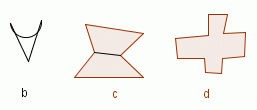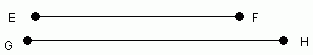Email us to get an instant 20% discount on highly effective K-12 Math & English kwizNET Programs!

#### Online Quiz (WorksheetABCD)

Questions Per Quiz = 2 4 6 8 10

### MEAP Preparation - Grade 7 Mathematics4.40 Geometry Review Test

 Q 1: What is the mid point of GH whose length is 11 cm?5.5 cm15 cm7.5 cm Q 2: If two lines are perpendicular to each other then all the pairs of adjacent angles formed are supplementary.TrueFalse Q 3: Which figure is concave polygon?bcd Q 4: The perimeter of a triangle with sides x, y and z is given by _________. x+y, y+z, z+xx + y + zxyz Q 5: Find which line segment is smaller.EF line segmentGH line segment Q 6: "The base angles of an isosceles triangle are congruent".FalseTrue Q 7: Find which line segment is smaller.IJ line segmentKL line segment Q 8: InABC, ĐA = 45°, ĐB = 90°, find ĐC.60°45°45°40° Question 9: This question is available to subscribers only! Question 10: This question is available to subscribers only!